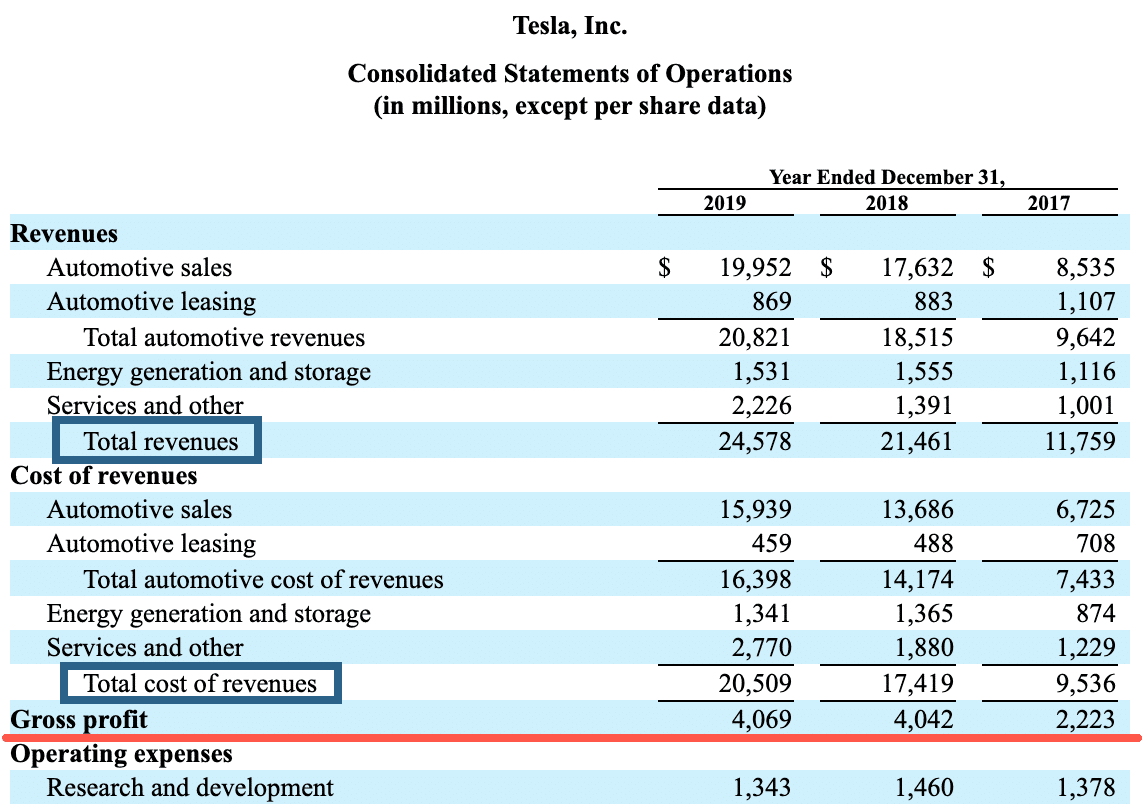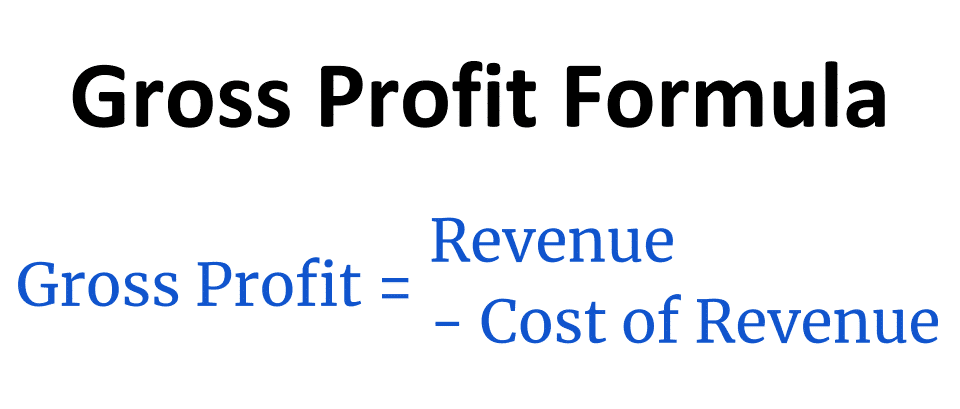# Gross Profit

Gross profit is a company's profit after subtracting the costs directly linked to making and delivering its products and services.

The formula for gross profit is calculated by subtracting the cost of goods sold (COGS) from the company's revenue.

Gross profit is often called gross income or gross margin.

Depending on the company, revenue may also be called "sales," and the cost of goods sold may be called "cost of revenue" or "cost of sales."

The revenue, cost of revenue, and gross profit are found on a company's income statement. Here's a screenshot of the 2019 income statement for Tesla (TSLA):As you can see, Tesla's revenue was \$24,578 million in 2019, with a cost of revenue of \$20,509. This gives a gross profit of \$24,578 - \$20,509 = \$4,069 million.

## Gross profit explained in plain English

For a business, revenue is the total amount of money made without accounting for any costs or expenses.

But gross profit tells you how much money is left after subtracting one major expense item from the revenue — the cost of goods sold.

But what is the cost of goods sold (COGS), and how is it different from other expenses?

The cost of goods sold is the added up cost of materials, labor, and other things that are variable based on the amount of product or service that the company makes.

The more product made or service rendered, the higher the cost of revenue.

Here are examples of the types of variable costs included in the cost of goods sold:

• Hourly labor
• Materials for production
• Equipment used in production
• Packaging
• Sales commissions
• Transaction fees on customer payments (including credit card fees)
• Shipping and fulfillment

Various other costs and expenses can be included if they are variable and directly related to the company’s output of products and services.

When all these variable costs are added up, the total amount is the cost of goods sold (or cost of revenue) used to calculate gross profit.

The amount of gross profit left after subtracting the cost of revenue tells you a lot about how efficiently the company runs.

It is preferable to see gross profit increase at the same rate as revenue. Or better yet, see it grow faster, which implies that the company is becoming more profitable.

## Gross profit vs. net profit

The cost of goods sold is different from operating expenses, which are fixed costs that do not directly depend on the company‘s output. These include rent, management salaries, marketing, insurance, and others.

When you subtract operating expenses from the gross profit, that number is the operating income. It is usually a much lower number than gross profit because it deducts other major expense items.

After subtracting all expenses, including so-called non-operating expenses like interest and taxes, what is left is net income (also called net profit or earnings).

Here’s how to calculate the three main levels of profit:

1. Gross Profit = Revenue - Cost of Revenue
2. Operating Income = Gross Profit - Operating Expenses
3. Net Profit = Operating Income - Other Costs

## Formula: how to calculate gross profitGross profit is one of the easiest financial metrics to calculate. The formula simply subtracts the cost of revenue from the revenue.

Formula: Gross Profit = Revenue - Cost of Revenue

For example, let’s imagine a coffee shop with \$200,000 in revenue (sales) per year.

They pay \$80,000 per year for their hourly staff and \$40,000 for goods like coffee beans and pastries. This is their cost of sales, amounting to \$120,000.

Then the company’s gross profit is \$200,000 - \$120,000 = \$80,000.

## Gross profit is not the same as gross margin

Although many people use the terms interchangeably, gross profit and gross margin are not the same.

Gross profit is a currency amount, while margin is a ratio or percentage. Gross profit margin is the percentage left as gross profit after subtracting the cost of revenue from the revenue.

You calculate it by dividing the gross profit by the revenue.

Formula: Gross Margin = Gross Profit / Revenue

In our coffee shop example above, the gross profit was \$80,000 from revenue of \$200,000.

This gives a gross profit margin of \$80,000 / \$200,000 = 0.4 = 40%.

For every dollar in sales, the coffee shop has 40 cents in gross profit that it can use to pay for other business expenses (and hopefully have something left as net profit at the end of the day).

The easiest way to remember the difference between profit and margin is:

• Profit / Income: Dollar amount (as in \$1,000)
• Margin: Percentage (as in 10%)

## A higher gross profit margin is better

If a company’s gross margin increases, it means that the company is making more money per unit sold. In other words, the company is becoming more efficient and generating more profits for the same amount of labor and material cost.

The best ways to increase gross margin are to raise prices or reduce the cost of producing the goods or services.

The gross margin is closely followed by investors and stock analysts, particularly for businesses with a high cost of revenue.

It is the single most important profitability metric for many companies and can make or break the difference between profits and losses.

A high gross margin can also imply that the company would be able to lower prices but still remain profitable. Having higher gross margins than direct competitors is a competitive advantage.

However, even if a company has high gross profit margins, it can still be unprofitable with a negative net profit margin. This often happens if operating expenses or other non-operating costs are high.

Some companies calculate separate gross profit and gross margin figures for different parts of their business.

For example, Apple (AAPL) had 31.6% gross margins on product sales in 2019, but 64% on its services business. This implies that the services business is more profitable for each dollar of revenue.Written by
Founder and CEO of Stock AnalysisEdited by
Head of Content at Stock Analysis